# 邻近汇总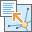“邻近汇总”工具通过直线距离或出行模式来查找输入层中要素指定距离内的要素。 然后，计算邻近要素的统计数据。

• 服务区(异步)
• 路径实用程序

## 工作流示意图## 用法说明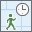步行时间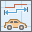农村行驶距离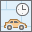行驶时间行驶距离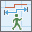步行距离农村驾驶时间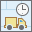货运时间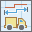货运距离

• 英里
• 英尺
• 千米

• 平方英里
• 平方千米
• 平方米
• 公顷
• 英亩

## 局限性

• 在其中测量距离的第一个输入图层的要素不能超过 1000 个。
• 仅当将点要素用作要在其中测量距离的输入图层时，才会启用出行模式。
• 步行时的行进时间不能超过 9 小时（540 分钟），所有其他出行时间不能超过 5 小时（300 分钟）。
• 步行时的距离不能超过 27 英里（43.45 千米），所有其他行驶距离不得超过 300 英里（482.8 千米）。
• 线和面是按比例进行汇总的；汇总线或面时，最好汇总绝对数据（如人口），而非相对数据（如平均收入）。
• 在使用出行模式时，如果工具执行时间超过 60 分钟，则会出现错误。 如果出现此错误，请尝试使用较少的输入要素重新运行分析。

## 邻近汇总的工作原理

### 等式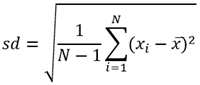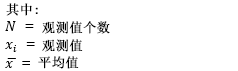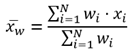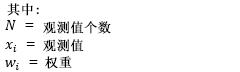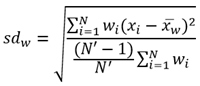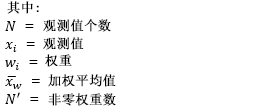### 点

``1 + 1 + 2 + 3 + 3 + 3 + 3 = 16``

``[1, 1, 2, 3, 3, 3, 3] = 1``

``[1, 1, 2, 3, 3, 3, 3] = 3``

``16/7 = 2.29``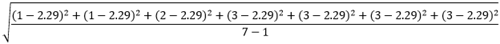``= 0.95``

### 线

``2 + 1 + 2 + 1 + 1 = 7``

``[2, 1, 2, 1, 1] = 1``

``[2, 1, 2, 1, 1] = 2``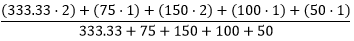``= 1.68``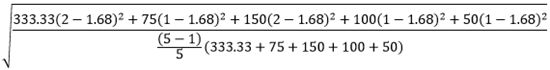``= 0.52``

### 面

``1 + 1 + 2 + 3 = 7``

``[1, 1, 2, 3] = 1``

``[1, 1, 2, 3] = 3````= 1.55``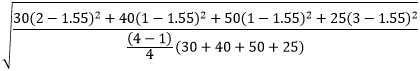``= 0.89``